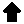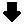Timing Calculation Monitor Timings into Absolute Pixels
General Timing Charts for monitors look like that:

 Ex.1: Related to Start of HSYNC / VSYNC = 0 ```Horizontal +-------------------+HDE |<------- D ------->| -----------+ +----------- ->| C |<- ->| E |<- | ---+ +---------------------------+ +--- HSPS ->| B |<- HSPE | | +---+ +---+ 0 |<------------- A ------------->|HT A = Total Hor. Frame duration in MicroSeconds 32.700 (us) B = Horizontal Sync Pulse duration 4.480 (us) C = Left Picture Border 2.360 (us) D = Active Picture Area 25.660 (us) E = Right Picture Border 0.200 (us) Vertical +-------------------+VDE |<------- R ------->| -----------+ +----------- ->| Q |<- ->| S |<- | ---+ +---------------------------+ +--- VSPS ->| P |<- VSPE | | +---+ +---+ 0 |<------------- O ------------->|VT O = Total Ver. Frame duration in MilliSeconds 16.650 (ms) P = Vertical Sync Pulse duration 0.065 (ms) Q = Upper Picture Border 0.816 (ms) R = Active Picture Area 15.672 (ms) S = Lower Picture Border 0.097 (ms) ```
 Ex.2: Related to Start Active Display Area = 0 ```Horizontal |<------------- A ------------->| 0 | | HT +-------------------+HDE +---- |<------- D ------->| | ---+ +-----------+ ->| E |<->| C |<- | | ---------------------------+ +----- HSPS ->| B |<- HSPE +---+ A = Total Hor. Frame duration in MicroSeconds 32.700 (us) B = Horizontal Sync Pulse duration 4.480 (us) C = Left Picture Border 2.360 (us) D = Active Picture Area 25.660 (us) E = Right Picture Border 0.200 (us) Vertical |<------------- O ------------->| 0 | | VT +-------------------+VDE +---- |<------- R ------->| | ---+ +-----------+ ->| Q |<->| S |<- | | ---------------------------+ +----- VSPS ->| P |<- VSPE +---+ O = Total Ver. Frame duration in MilliSeconds 16.650 (ms) P = Vertical Sync Pulse duration 0.065 (ms) Q = Upper Picture Border 0.816 (ms) R = Active Picture Area 15.672 (ms) S = Lower Picture Border 0.097 (ms) ```

The two sets of graphics above are similar to what you may find in your monitors' manual or (in tabellaric form) on the manufacturers support pages. Sometimes these values are hidden behind some strange "slang" but if they are accompanied by a picture like these above you will be able to get out the timing values.
I would recommend to write them into a table and use the letters A - S to name the rows of this table. It makes it a bit easier to track the following explanations :-)

As you may have mentioned: the letters for the signal timings are the same in both of the above examples - only the positions changed. Basically there are two methods where to start with the deflection timings: 0/0 is set at the beginning of the Sync-signals (Ex. 1) or 0/0 is set at the top / left corner of the Active Display Area (technoslovakian for "the picture you see on the screen") - like in Ex. 2.
Both objections are identical - and so are the used values. It is only a matter of the point of view how to look at them. Don't get confused by that.

It is only a bit complicated to re-calculate the pixel values required for the Linux ModeLine from out of these values.

How can that be done ?

A bit of Theory. (Darn !)

To recalculate the "Horizontal Pixel Frequency" in KHz you only need to divide 1 through the Total Horizontal Duration (A above) and multiply it by 1000 to get the (proper dimensioned) frequency from the time.
Assumed the horizontal total is 32.700 us you will get a value of 30.58 KHz.

1 / 32.700 us * 1000 = 30.581 KHz

To recalculate the vertical refresh frequency -known as "pictures per second"- you also only need to divide 1 through the Total Vertical Duration (O in the graphic) and multiply it by 1000.
Assumed it is 16.650 ms you will get out 60.06 Hz vertical refresh.

1 / 16.650 ms * 1000 = 60.060 Hz

To get out the Horizontal total pixels is a bit more trickier. Assumed you want to set a resolution of 640 x 480 in that mode, you need to divide the duration for the Active Picture Area (D in the above scheme) of 25.660 us through 640 pixels. This results in 0.04 us / pixel. Now calculate the 3 values B, C and E through 0.04 and add them to 640:

4.480 us / 0.04 us-pixel = 112 pixel
2.360 us / 0.04 us-pixel = 59 pixel
0.200 us / 0.04 us-pixel = 5 pixel

640 + 112 + 59 + 5 = 816 pixel total horizontal.

Alternatively you can divide the horizontal Total value A through 0.04 us/pixel:

32.700 us / 0.04 us-pixel = 816

A difference of +/- 0.5 pixels is caused by inaccuracies during measuring and calculating the values for B, C and E. It *might* be more accurate to use this value instead of the sum (B+C+E) + 640 pixel.

To get out the Vertical total lines the calculation is the same. First you divide the Active Picture vertical value (R in the above) through 480 and get ms per line. Then you divide the values for P, Q and S and add the lines to 480.

15.672 ms / 480 lines = 0.03265 ms-line

0.065 ms / 0.03265 ms-line = 2 lines
0.816 ms / 0.03265 ms-line = 25 lines
0.097 ms / 0.03265 ms-line = 3 lines

480 + 2 + 25 + 3 = 510 lines total vertical.

Here you can also alternatively divide the Vertical Total through the 0.03264 ms-line:

16.650 ms / 0.03265 ms-line = 510 lines

Remark: if the divisions for horizontal and vertical pixels / lines leads to rather odd values that do not round close to the next integer - the mode will most likely not work with the choosen resolution. You must come out on values that round about to the next integer. 1.98 and 2.05 will most likely round to 2, while 1.55 and 2.45 will not very well. Try another resolution.

We have now:
• Horizontal total pixel
• Vertical total lines
• Horizontal frequency
• Vertical frequency

From out of Horizontal and Vertical totals and Vertical Refresh we can calculate the Pixel Clock, by simply multiplying them.

816 pixels * 510 lines * 60.06 Hz refresh = 24.995 MHz pixelclock.

A Pixel Clock setting of straight 25.00 MHz will do fine here.

Remark: The XGA-2 system is an almost free-programmable system, but it has its limits. I would not recommend to use a pixel-clock above 77MHz - even though the XGA-2 RAMDAC is specified to 90MHz. The pictures will be rather distorted and speckled.
In addition there are "divider steps". From 16.25 - 32.00 MHz the steps are 0.25MHz, from 32.50 to 64.00 MHz the steps are 0.50 MHz and from 65.00 to (theoretical) 128.00 MHz the steps are 1.00 MHz.
If your calculation ends up in 49.48 MHz pixel-clock - use 49.50 MHz (0.50 MHz steps in this range). If it ends up in 25.21 MHz you should use 25.25MHz (0.25MHz steps in this range).
You may however ignore these "unmatching" values and insert them into your ModeLine - and the XF86 Driver will round them up or down accordingly. At least I haven't found any problems in using even oddest values for the pixel-clock.

Different Approach in Linux

The Linux ModeLine however does not fiddle with timings in microseconds and milliseconds. It uses basically 10 parameters, from which the 8 "resolution-dependent" parameters are relative from the point 0,0 (horizontal / vertical) which is the start of the active display area:

ModeLine "xxxxxx" pixelclock screen_width hsync_start hsync_end h_total screen_height vsync_start vsync_end v_total [optional parameters]

Example:

ModeLine "640x480" 25.00 640 645 757 816 480 483 485 510 +hsync +vsync

How to calculate these values ?

Pretty easy - because we have the required values already and only need to re-arrange them.
The 640 and 480 are obvious - they are the horizontal and vertical pixels of the active picture area.
The 645 is the 640 pixel + the right border (E) to the point where the horizontal sync signal starts. Add D and E for this value.
The 757 is the Hsync End - 640 pixels of the picture, 5 pixels of the right border and 112 pixels of the Sync signal duration. Simply add the pixel values for D, E and B.
The 816 is our Horizontal Total again.

For the vertical values you only need to add the 3 lines for the lower border (S) to the 480 and get 483. Add the 2 lines for the Vertical Sync Signal (P) and you get the 485. The 510 is our Vertical Total again.

The settings for the HSync and VSync polarity need to be obtained from the monitors papers. As a guideline you can assume that the 640 x 400 mode uses -Hsync and +Vsync, the 640 x 480 modes mainly use +Hsync and +Vsync and all higher modes (800 x 600 and above) mainly use -Hsync and -Vsync polarities.

Want it easier ?

 Well then click on the Start button below and use my Timing Calculator ! However: this requires a JAVASCRIPT-enabled Browser.Go to MCA-IndexVisit my Homepage

© 1999 by Peter H. Wendt